Home MATHEMATICS TOPIC 3: DECIMAL AND PERCENTAGE ~ MATHEMATICS FORM 1

# TOPIC 3: DECIMAL AND PERCENTAGE ~ MATHEMATICS FORM 1

1060
0
SHAREThe Concept of Decimals
Explain the concept of decimals
A decimal- is defined as a number which consist of two parts separated by a point.The parts are whole number part and fractional part
Example 1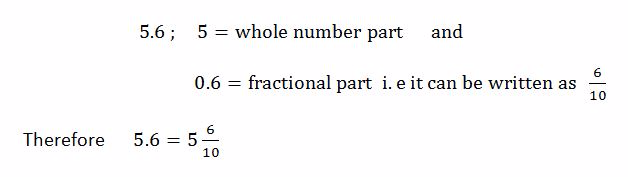Example 2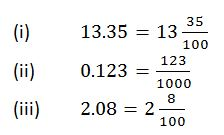Conversion of Fractions to Terminating Decimals and Vice Versa
Convert fractions to terminating decimals and vice versa
The first place after the decimal point is called tenths.The second place after the decimal point is called hundredths e.t.c
Consider the decimal number 8.152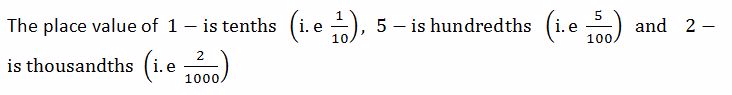NOTE
• To convert a fraction into decimal, divide the numerator by denominator
• To
convert a decimal into fraction, write the digits after the decimal
point as tenths, or hundredths or thousandths depending on the number of
decimal places.
Example 3
Convert the following fractions into decimals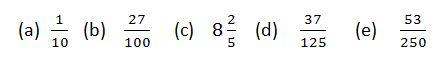Solution
Divide the numerator by denominator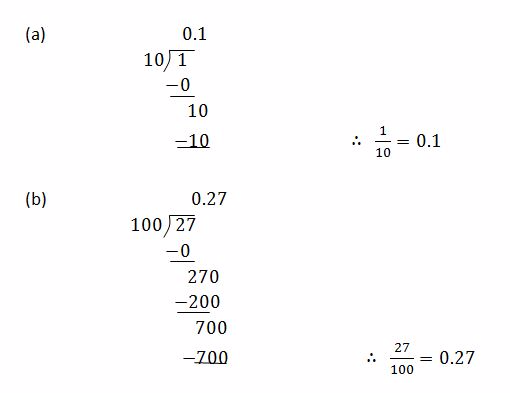#### 1 COMMENT

1.Benadetha Mwinuka

Good job